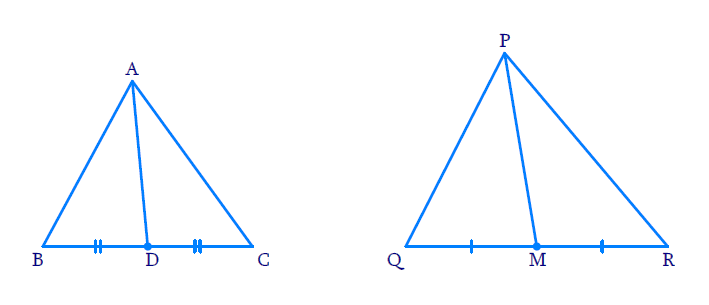# Ex.6.3 Q12 Triangles Solution - NCERT Maths Class 10

Go back to  'Ex.6.3'

## Question

Sides $$AB$$ and $$BC$$ and median $$AD$$ of a $$\triangle ABC$$ are respectively proportional to sides $$PQ , QR$$ and median $$PM$$ of $$\Delta PQR$$ (see the below Figure) .

Show that $$\Delta ABC$$~ $$\Delta PQR$$.

DiagramVideo Solution
Triangles
Ex 6.3 | Question 12

## Text Solution

Reasoning:

As we know if one angle of a triangle is equal to one angle of the other triangle and the sides including these angles are proportional, then the two triangles are similar.

This is referred as $$SAS$$ (Side–Angle–Side)  criterion for two triangles.

Steps:

In $$\Delta ABC$$ and $$\Delta PQR$$

\begin{align} \frac{AB}{PQ}=\frac{BC}{QM}=\frac{AD}{PM} \,\,\,\text{[Given]} \\ \end{align}

$$\because$$ AD  and PM are median of $$\Delta ABC$$ and $$\Delta PQR$$ respectively

\begin{align}&\Rightarrow \frac{BD}{QM}=\frac{{\frac{{BC}}{2}}}{{\frac{{QR}}{2}}}={\frac{BC}{QR}} \end{align}

Now In $$\Delta \rm{ABD}\,\,\text {and}\,\Delta \rm{PQM}$$

\begin{align}&\frac{AB}{PQ}=\frac{BD}{QM}=\frac{AD}{PM} \\ \end{align}

$\Rightarrow \Delta \rm{A B D} \sim \Delta \rm{P Q M}$

Now in $$\Delta \rm{A B C}\,\text{and}\, \Delta \rm{P Q R}$$

\begin{align}&\frac{A B}{P Q}=\frac{B C}{Q R}\\&(\text {Given in the statement}) \\ &\angle A B C=\angle P Q R\\&[\because \Delta A B D\sim\Delta P Q M] \\ \Rightarrow\! \Delta A B C &\!\sim \! \Delta P Q R \,\,[\text{SAS} \text { Criterion }\!\!]\end{align}

Learn from the best math teachers and top your exams

• Live one on one classroom and doubt clearing
• Practice worksheets in and after class for conceptual clarity
• Personalized curriculum to keep up with school